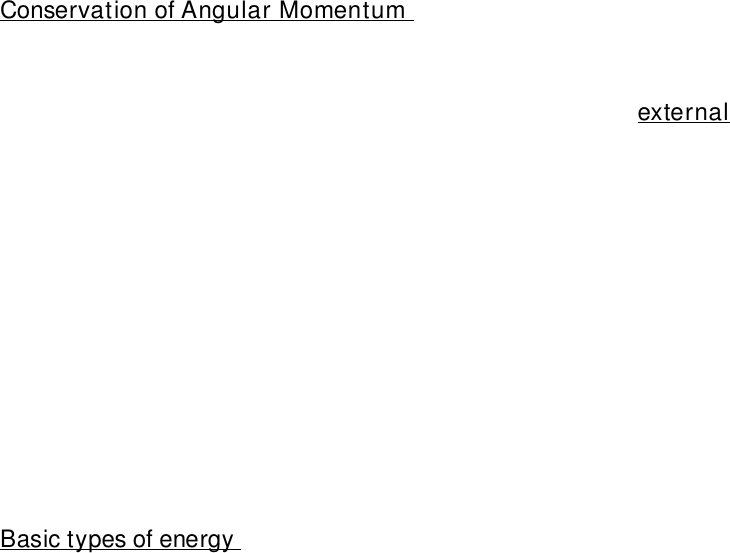Class Notes (1,100,000)
CA (640,000)
UTSG (50,000)
AST (700)
AST201H1 (200)
Lecture

# conservation laws and energy from lecture 3

Department
Astronomy & Astrophysics
Course Code
AST201H1
Professor
Stefan Mochnacki

Page:
of 2Lecture 3- pt 2
Conservation Laws in Astronomy
The total momentum of interacting objects cannot change unless an external
force is acting on them
Interacting objects exchange momentum through equal and opposite forces
Conservation of Angular Momentum
-angular momentum= mass x velocity x radius
angular momentum of an object cannot change unless an external twisting force
is acting on it thus, angular momentum stays constant
ex: increase of radius= decrease of velocity
earth experiences no twisting force as it orbits the sun so orbits and rotation will
continue indefinitely (exception: tidal force with moon)
Energy
-energy makes matter move
-energy is conserved but it can transfer, convert
-total energy: kinetic and potential
Basic types of energy
Kinetic (motion)
Stored or potential
*energy can change type but cannot be created or destroyed [conservation law of
energy]
Thermal Energy
Collective kinetic energy of many particles
Ex: in a rock, in air, in water
Thermal energy is related to temperature but it is NOT the same
Temperature is the average kinetic energy of the many particles in a substance
*lower amount of particles, lower temperature, less movement
-KELVIN, CELSIUS, FAHRENHEIT
www.notesolution.com
Thermal energy is a measure of the total kinetic energy of all the particles in a
substance
therefore depends on both temperature and density
Gravitational Potential Energy
On earth: depends on
Objects mass (m)
Strength of gravity (g)
Distance object could potentially fall
*total energy is the same at all points in the balls flight, fall
*more gravitational potential energy-less thermal energy
in space: an object or gas cloud has more gravitational energy when it is spread
out than when it contracts
a contracting cloud converts gravitational potential energy to thermal energy
contraction and energy conversion, results: hot accretion disk + star
Mass-Energy
Mass itself is a form of potential energy
E=MC^2 Einstein, 1905
E: energy converted from mass loss /
M: mass difference
C: speed of light
Concentrated energy can spontaneously turn into particles
Small amount of mass can release a great deal of energy
Radiation was the dominant mass in the universe- after the Big Bang
www.notesolution.com